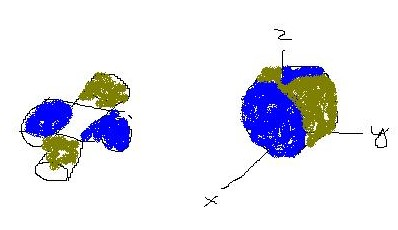# The figure shows the surface created when the cylinder y^2+Z^2=1 intersects the cylinder x^2+Z^2=1. Find the area of this surface.The figure is something like:FSP02510400011.jpgFSZsodni3 2020-12-16 Answered

The figure shows the surface created when the cylinder ${y}^{2}+{Z}^{2}=1$ intersects the cylinder ${x}^{2}+{Z}^{2}=1$. Find the area of this surface.
The figure is something like:You can still ask an expert for help

• Questions are typically answered in as fast as 30 minutes

Solve your problem for the price of one coffee

• Math expert for every subject
• Pay only if we can solve itstuth1
Note that the given cylinder will intersect at four congruent surfaces. e. equal in area. So we need to find the area of only one surface and then multiply it by 4.
First, we find the area of the face of the surface that intersect the positive y axis. So the surface lies above the region ${x}^{2}+{z}^{2}=1$ then $z=f\left(x,z\right)=\sqrt{1-{z}^{2}}$
$=\int {\int }_{{x}^{2}+{z}^{2}\le 1}\sqrt{{f}_{x}^{2}+{f}_{z}^{2}+1}dA$
$=\int {\int }_{{x}^{2}+{z}^{2}\le 1}\sqrt{\frac{{z}^{2}}{1-{z}^{2}}+1}dA$
$={\int }_{-1}^{1}{\int }_{-\sqrt{1-{z}^{2}}}^{\sqrt{1+{z}^{2}}}\frac{1}{\sqrt{1-{z}^{2}}}dxdz$
$=4{\int }_{0}^{1}{\int }_{0}^{\sqrt{1-{z}^{2}}}\frac{1}{\sqrt{1-{z}^{2}}}dxdz$
$=\underset{t\to 1}{lim}+4{\int }_{0}^{t}{\int }_{0}^{\sqrt{1-{z}^{2}}}\frac{1}{\sqrt{1-{z}^{2}}}dxdz$
$=\underset{t\to 1}{lim}+4{\int }_{0}^{t}1dt$
$=4$
Hence the total area of the intersected surface is $4×4=16$